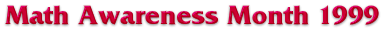```

```# Poiseuille's Law: Graph Exploration of Radial Dependence

We show next the graph of the power law relation between flow and diameter (and, equivalently, radius).With all of the parameters except the diameter fixed, choose the diameter that you want. You can determine the value of the flow geometrically by finding your diameter value along the horizontal axis (independent variable), finding the point on the graph directly above this number, and then reading the value of the number directly to the left of this point (dependent variable).

This graph is typical of a power law. Similar to the linear relation for pressure, this has an increasing graph. If the diameter gets arbitrarily large, then the flow does as well. Also, as the diameter gets smaller, the flow decreases. This corresponds to the physically meaningful result that no fluid can flow through a closed tube. It is very important that the shape of this curve is convex (curved upward). This results in the valuable sensitivity of the flow to the size of the diameter. Physiologically, this is the most important factor in controlling the rate blood flows through your body.

M & B Home | Previous | Next Subcategory | Next Category

D. Brian Walton is supported by a National Science Foundation Graduate Research Fellowship.